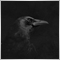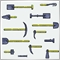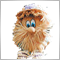# Get value from iBarShift(). - page 21056

I have done that. thanks.1131

"Return Value

The index of the bar corresponding to the specified time. If the bar corresponding to the specified time is not found (there is a gap in the history), the function returns -1 or the index of the nearest bar (depending on the 'exact' parameter)."

Did you try setting the exact parameter to false?1056

Tobias Johannes Zimmer #:
"Return Value

The index of the bar corresponding to the specified time. If the bar corresponding to the specified time is not found (there is a gap in the history), the function returns -1 or the index of the nearest bar (depending on the 'exact' parameter)."

Did you try setting the exact parameter to false?

Yes, it is false, I did not change it since that is the default value.Moderator
301933

An example of how to work in the indicator using 'iBarShift' when requesting data from someone else's timeframe is in the indicator.

The main thing: it is always necessary to check - what did the function return? Is there an error?

```   for(int i=limit; i<rates_total; i++)
{
int index_tf_0=iBarShift(Symbol(),Inp_TF_0,time[i],false);
if(index_tf_0<0)
return(0);
double price_tf_0=iClose(Symbol(),Inp_TF_0,index_tf_0+1);
ClosePriceTF0Buffer[i]=price_tf_0;

int index_tf_1=iBarShift(Symbol(),Inp_TF_1,time[i],false);
if(index_tf_1<0)
return(0);
double price_tf_1=iClose(Symbol(),Inp_TF_1,index_tf_1+1);
ClosePriceTF1Buffer[i]=price_tf_1;

MedianPriceBuffer[i]=(price_tf_0+price_tf_1)/2.0;
}```
Files:1056